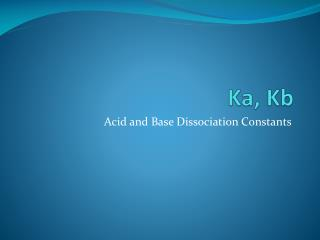DownloadDownload PresentationKa, Kb

Ka, Kb

Télécharger la présentationKa, Kb

- - - - - - - - - - - - - - - - - - - - - - - - - - - E N D - - - - - - - - - - - - - - - - - - - - - - - - - - -
Presentation Transcript

1. Ka, Kb Acid and Base Dissociation Constants

2. How do we calculate [H+] for a weak acid? • We know that strong acids dissociate 100% and that, therefore, the [H+] equals that [acid] that we start with • What about weak acids? • Don’t ionize 100%, so the [H+] is NOT the same as our starting concentration of our acid!

3. Recall Kw • Autoionization of water: H2O ↔ H+ + OH- Or H2O + H2O ↔ H3O+ + OH- • Keq = • Kw = [H3O+] [OH-] = 1.0 x 10-14

4. Ka • Similarly to Kw we can write an equilibrium expression for the dissociation of a weak acid • The equilibrium for a weak, monoprotic acid (HA) looks like this: HA(aq) + H2O(l)↔ H3O+(aq) + A-(aq) • So, we can write an equilibrium expression that looks like this: where Ka is the acid ionization constant

5. Ka • Example: Write the equilibrium expression for the ionization of acetic acid. CH3COOH(aq) ↔ CH3COO-(aq) + H+(aq)

6. Ka and Acid Strength • Ka values are typically between 1 – 1 x 10-16 • The higher the value of Ka, the more the acid dissociates in water and, hence, the stronger the acid

7. What about weak bases? • Weak bases also form an equilibrium in water: B(aq) + H2O(l) ↔ HB+(aq) + OH-(aq) • This can be represented by the base dissociation constant: • Like Ka, a higher Kb means that more B has dissociated and, therefore, the stronger the base

8. Note: Coefficients and Equilibrium Expressions • If you have coefficients in your reaction equation, they become subscripts in the equilibrium expression: 2AB → A2 + B2

9. Try it • Write the equilibrium expression for the dissociation of NH3 in water. NH3(aq) + H2O(l) ↔ NH4(aq)+ + OH-(aq) Try the Self Test 10.2

10. So how does Ka help us find the [H+]? • The Ka’s for almost every weak acid you could think of have been measured (at 25oC) and recorded • If we know the value of Ka and the starting concentration of our weak acid, we can solve for [H+]

11. Try It: • What is the concentration of H+ in 0.50M HF at 25oC? • From the acid table, Ka = 7.1 x 10-4, so: HF(aq) ↔ H+(aq) + F-(aq) • Now what? Now, we use ICE tables!

12. ICE table • HF(aq) ↔ H+(aq) + F-(aq)

13. Solve for x • In this case x is our [H+]

14. Short Cut: • If < 500, the change in the initial concentration (x) is negligible and can be ignored. ]

15. Percent Dissociation (aka. Percent Ionization) • The fraction of molecules that dissociate compared to the initial concentration, expressed as a percent: • Percent dissociation = • Ex: If a 0.10 M solution of benzoic acid was found to dissociate to give a [H+] = 1.1 x 10-3 M, the percent dissociation would be: